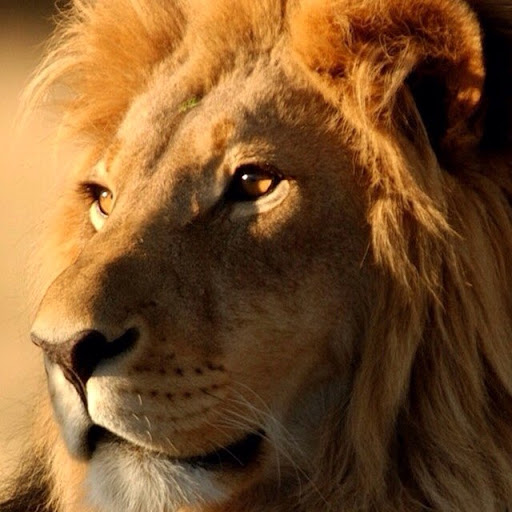JReese82Joined: Jul 11, 2017
• Posts: 1
July 11th, 2017 at 8:04:31 AM permalink
My question is referring to standard six sided dice. Is the probability of rolling three of a specific kind the same as rolling four of any kind? Just in case I'm not being clear, if you rolled three dice would your probability of getting three sixes be the same as rolling four dice all with the same number. Finally would this trend continue so that rolling four of a specific kind have the same probability of rolling five of any kind and so on?
OnceDearJoined: Jun 1, 2014
• Posts: 4211
July 11th, 2017 at 9:07:15 AM permalink
Quote: JReese82

My question is referring to standard six sided dice. Is the probability of rolling three of a specific kind the same as rolling four of any kind? Just in case I'm not being clear, if you rolled three dice would your probability of getting three sixes be the same as rolling four dice all with the same number. Finally would this trend continue so that rolling four of a specific kind have the same probability of rolling five of any kind and so on?

Let's run the numbers.. . . .
The same maths applies whether I roll one die three times or whether I roll three dice at the same time.
So, we will assume it's a single die.
The probability that my next three rolls of that die will all land as 1 will be (1/6)*(1/6)*(1/6) = 1/216
Same probability for rolling 3 2's or 3 3s or etc up to .... 3 6s
So probability that the next three rolls will be of the same (unstated) value will be the sum of those 6 probabilities =6/216=1/36

Now, if we do the same exercise for 4 rolls

The probability that my next four rolls of that die will all land as 1 will be (1/6)*(1/6)*(1/6)*(1/6) = 1/1296
Same probability for rolling 4 2's or 4 3s or etc up to .... 4 6s
So probability that the next four rolls will be of the same (unstated) value will be the sum of those 6 probabilities =6/1296=1/216

So yes, the probability of rolling three sixes is the same as rolling four unstated but same values: An interesting observation.
Similarly the probability of rolling four sixes is the same as rolling five unstated but same values: Another interesting observation.
And yes: The pattern continues, if that is indeed what you meant.

Now I must sob at the thought that 'at least one of the dice was a two' (inside joke)
Last edited by: OnceDear on Jul 11, 2017
If you are enjoying the game, you're already winning.
AyecarumbaJoined: Nov 17, 2009
• Posts: 6689
July 11th, 2017 at 9:11:02 AM permalink
No. The probability of rolling a specific number on each die is 1/6. The probability of rolling two of the same specific number is (1/6)*(1/6); the probability of rolling three of the same number is (1/6)*(1/6)*(1/6), and so on.

Edit: I see OD clarified what "the same number" means in the original question. Interesting.
Simplicity is the ultimate sophistication - Leonardo da Vinci
IbeatyouracesJoined: Jan 12, 2010
• Posts: 11933
July 11th, 2017 at 9:14:38 AM permalink
It would get harder as you add in more dice to roll all of a kind.
DUHHIIIIIIIII HEARD THAT!
DocJoined: Feb 27, 2010